In :
from __future__ import division
%pylab inline

Populating the interactive namespace from numpy and matplotlib


$\newcommand{\dotp}{\langle #1, #2 \rangle}$ $\newcommand{\umin}{\underset{#1}{\min}\;}$ $\newcommand{\RR}{\mathbb{R}}$ $\newcommand{\pd}{ \frac{ \partial #1}{\partial #2} }$ $\newcommand{\norm}{\|#1\|}$ $\newcommand{\pa}{\left(#1\right)}$

We consider the problem of finding a minimizer of a convex smooth function $\;f : \RR^d \rightarrow \RR$; that is, we want to solve $$\umin{x \in \RR^d} f(x)$$

Note that the minimizer is not necessarily unique.

The simplest method is gradient descent, which iteratively computes $$x^{(k+1)} = x^{(k)} - \tau \nabla f(x^{(k)}),$$ where $\nabla f(x) \in \RR^d$ is the gradient of $f$ at $x$, $x^{(0)} \in \RR^d$ is an arbitrary initial point, and the stepsize $\tau$ must satisfy $$0<\tau<2/\beta,$$ to have convergence, where $\beta$ is a Lipschitz constant of $\nabla f$; that is, $$\forall (x,x') \in \RR^N \times \RR^N, \quad \norm{\nabla f(x) - \nabla f(x')} \leq \beta \norm{x-x'}.$$

For instance, if $f$ is of class $C^2$, $$\beta= \sup_x \norm{Hf(x)},$$ where $Hf(x) \in \RR^{d \times d}$ is the Hessian of $f$ at $x$ and $\norm{\cdot}$ is the spectral operator norm (largest eigenvalue).

We consider a simple problem, corresponding to the minimization of a 2-D ($d=2$) quadratic form $$f(x) = \frac{1}{2} \pa{ x_1^2 + \eta x_2^2 } ,$$ where $\eta>0$ controls the anisotropy, and hence the difficulty, of the problem.

We can note that minimizing a strongly convex quadratic function is equivalent to solving a positive definite linear system. Gradient descent is then equivalent to the Richardson iteration applied to this linear system.

We define the anisotropy parameter $\eta$:

In :
eta = 8


We define the function $f$:

In :
f = lambda x : (x**2 + eta*x**2) / 2


Note: a 'lambda' function is a one-line function definition; in Matlab, this would be [email protected](x)(x(1)^2+eta*x(2)^2)/2;

An example of function evaluation:

In :
f([2,3])

Out:
38.0

We display the function using a contourplot:

In :
figsize(6,6)
t = linspace(-.7,.7,101)
[u,v] = meshgrid(t,t)
F = (u**2 + eta*v**2) / 2
contourf(t,t,F,35)

Out:
<matplotlib.contour.QuadContourSet instance at 0x10853c9e0>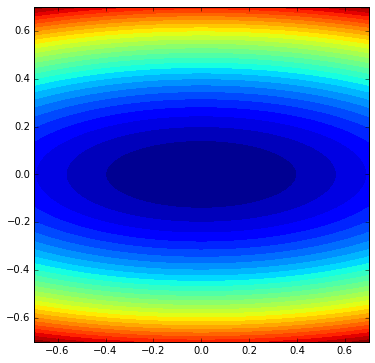We define the gradient of $f$:

In :
Grad_f = lambda x : array([x, eta*x])


An example of evaluation:

In :
Grad_f([1,2])

Out:
array([ 1, 16])

Since $f$ is quadratic, its Hessian is the constant matrix $\left(\begin{array}{cc}1&0\\0&\eta\end{array}\right)$. Its spectral norm, which is the Lipschitz constant of Grad_f, is $\beta=\max(1,\eta)$.

The stepsize $\tau$ must satisfy $0< \tau < 2/\beta$.

In :
tau = 1.8/max(eta,1); tau

Out:
0.225

Now we implement the gradient descent method: given the initial estimate $x^{(0)}$ of the solution, the stepsize $\tau$ and the number $k$ of iterations, we compute $x^{(k)}$:

In :
nbiter = 10
x = [1,1]  # initial estimate of the solution
for iter in range(nbiter):  # iter goes from 0 to nbiter-1
x   # to display x, like in Matlab. Use print(x) if this is not the last command of the cell, else nothing is displayed

Out:
array([ 0.07816584,  0.10737418])

Note: there is no 'end' for the loops in Python; instead, the content of the loop is determined by the indentation: here four blank spaces before x=...

In :
f(_) # _ is the current variable, like Matlab's ans

Out:
0.049171809817584886

We encapsulate the code in a function called GradientDescent.

In :
def GradDescent(Grad_f, x0, nbiter, tau):
x = x0;
for iter in range(nbiter):  # iter goes from 0 to nbiter-1
x = x - tau*Grad_f(x)   # x has type 'array'. Like in C, one can also write x-=tau*Grad_f(x)
return x

In :
GradDescent(Grad_f,[1,1],10,tau)

Out:
array([ 0.07816584,  0.10737418])

We define a function called GradDescentArray which puts the iterates in a 'matrix' (a 2-D array in fact); they are first put in a list and the list is converted to an array at the end (the + operation on lists concatenates them, whereas on arrays this is the classical elementwise addition).

In :
def GradDescentArray(Grad_f, x0, nbiter, tau):
x = x0;
xlist=[list(x0)];  # the cast is just in case x0 would be an array
for iter in range(nbiter):
xlist = xlist + [list(x)]
return array(xlist)

In :
xarray=GradDescentArray(Grad_f,[0.6,0.6],10,0.225)
xarray

Out:
array([[ 0.6       ,  0.6       ],
[ 0.465     , -0.48      ],
[ 0.360375  ,  0.384     ],
[ 0.27929063, -0.3072    ],
[ 0.21645023,  0.24576   ],
[ 0.16774893, -0.196608  ],
[ 0.13000542,  0.1572864 ],
[ 0.1007542 , -0.12582912],
[ 0.07808451,  0.1006633 ],
[ 0.06051549, -0.08053064],
[ 0.04689951,  0.06442451]])

We can access the elements of an array like in Matlab. Be careful: like in C, the first element has index 0! Some examples:

In :
xarray[1,0]

Out:
0.46499999999999997
In :
xarray[1,:]

Out:
array([ 0.465, -0.48 ])
In :
xarray[:,0]

Out:
array([ 0.6       ,  0.465     ,  0.360375  ,  0.27929063,  0.21645023,
0.16774893,  0.13000542,  0.1007542 ,  0.07808451,  0.06051549,
0.04689951])

We can apply the function $f$ to every iterate $x^{(k)}$ of the list xarray, using the command map:

In :
map(f,xarray)

Out:
[1.6199999999999999,
1.0297125000000003,
0.65475907031250036,
0.41648898660644562,
0.26501726238049639,
0.16868867468928564,
0.10740675137733219,
0.06840757437694138,
0.043580991731946392,
0.027771796276949725,
0.017701851534330564]

We plot the cost function $f(x^{(k)})$ as a function of $k$, in log-scale:

In :
plot(range(len(xarray)),log10(map(f,xarray)),'o-')

Out:
[<matplotlib.lines.Line2D at 0x1086b7b50>]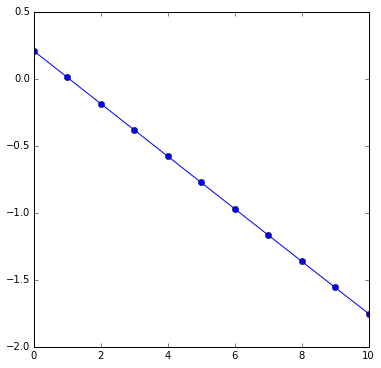We remark that because the function $f$ is strongly convex, the convergence is linear. Also, we can note that gradient descent is monotonic: $f(x^{(k)})$ is decreased at every iteration.

We plot the iterates above the contourplot of $f$:

In :
figsize(8,8)
contourf(t,t,F,35)
plot(xarray[:,0], xarray[:,1], 'w.-')

Out:
[<matplotlib.lines.Line2D at 0x1062a1b90>]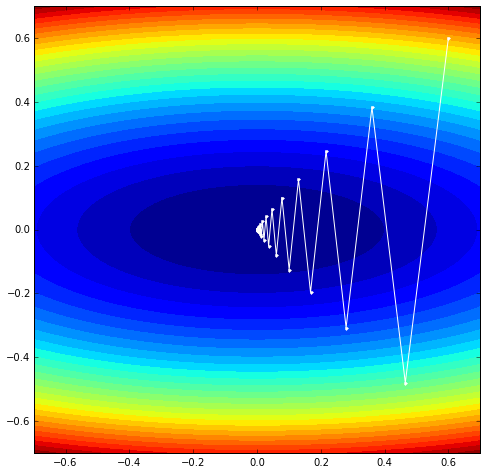## Gradient descent in large dimension: image restoration¶

Local differential operators like the discrete gradient are fundamental for variational image processing.

We load the image Lena in the array $x^\sharp$ (we choose the symbol # - 'sharp', because the image is clean, not degraded).

In :
from scipy import misc
xsharp = misc.lena()
print(xsharp.shape) # like Matlab's size(xsharp). Given as a tuple.
print("The size of the image is %s x %s." % (xsharp.shape,xsharp.shape))
print("The range of the pixel values is [%s,%s]." % (xsharp.min(),xsharp.max()))
xsharp = xsharp.astype(float32)  # like Matlab's xsharp=double(xsharp) so that the pixel values are floating point numbers

(512, 512)
The size of the image is 512 x 512.
The range of the pixel values is [25,245].


We display the image.

In :
figsize(11,11)
imshow(xsharp, interpolation='nearest', cmap=cm.gray, vmin=0, vmax=255)
# Without specifying vmin and vmax, imshow auto-adjusts its range so that black and white are
# the min and max of the data, respectively, like Matlab's imagesc.
colorbar()       # displays the color bar close to the image
#axis('off')     # uncomment to remove the axes
title('This is Lena')

Out:
<matplotlib.text.Text at 0x10958b1d0>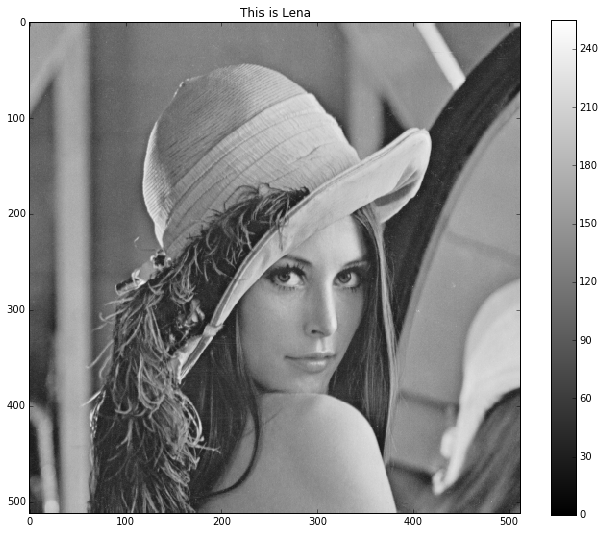We define the 'discrete gradient' $D$, which is the linear operator which maps an image to an image whose values are pairs of vertical and horizontal finite differences:

$$(D x)_{n_1,n_2} = \big((D x)_{n_1,n_2,v},(D x)_{n_1,n_2,h}\big)=( x_{n_1+1,n_2}-x_{n_1,n_2}, x_{n_1,n_2+1}-x_{n_1,n_2} ) \in \RR^2,$$

where $n_1$ and $n_2$ are the row and column numbers, respectively (the origin $n_1=n_2=0$ corresponds to the pixel at the top left corner) and where Neumann boundary conditions are assumed: a difference accross the boundary is zero.

Let us define the operator $D$ as a function:

In :
def D(x):
vdiff = r_[diff(x,1,0), zeros([1,x.shape])] # the r_ command concatenates along the rows
hdiff = c_[diff(x,1,1), zeros([x.shape,1])] # the c_ command concatenates along the columns
return concatenate((vdiff[...,newaxis], hdiff[...,newaxis]), axis=2) # combination along a third dimension
# An alternative, more compact, definition:
#D = lambda x : c_['2,3',r_[diff(x,1,0), zeros([1,x.shape])],c_[diff(x,1,1), zeros([x.shape,1])]]

In :
v = D(xsharp)


We display the two components as two grayscale images.

In :
from mpl_toolkits.axes_grid1 import make_axes_locatable
fig, (axv,axh) = subplots(1,2,figsize=(16,7)) # one figure with two horizontal subfigures
suptitle('The two images of vertical and horizontal finite differences')
imv=axv.imshow(v[:,:,0], cmap=cm.gray)
imh=axh.imshow(v[:,:,1], cmap=cm.gray)
axv.set_title('Image of vertical finite differences')
axh.set_title('Image of horizontal finite differences')
dividerv = make_axes_locatable(axv)
colorbar(imv,cax=caxv)
dividerh = make_axes_locatable(axh)
colorbar(imh,cax=caxh)

Out:
<matplotlib.colorbar.Colorbar instance at 0x10bd0ff80>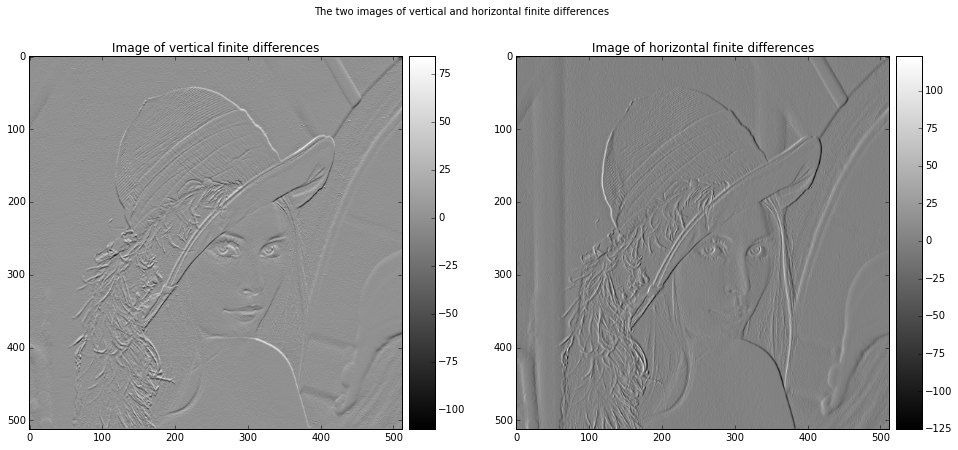Let us display the image of the magnitudes $\norm{(D x)_{n_1,n_2}}$, which are large near edges.

In :
figsize(11,11)
imshow(sqrt(sum(v**2,2)), cmap=cm.gray, vmin=0)
colorbar()